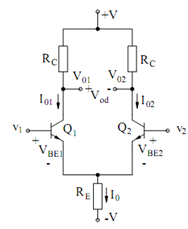## Differential Amplifier Assignment Help

Assignment Help: >> Transistor Amplifier Circuits - Differential Amplifier

Differential Amplifier:

The function of a differential amplifier (DA) is to amplify the difference among two signals. Such an amplifier is required in many instrumentation and measurement systems, the most common instance being in detecting balance in bridge measurements. The DA also forms the input stage of several analog integrated circuits and is  significant building block of IC operational amplifiers.

Figure illustrates the circuit diagram of a differential amplifier with v1 and v2 as the input signals and vo as the output signal. Ideally, we must have

V0 = Ad (v1 - v2)

where the subscript d on the gain parameter A signifies 'differential'. In a practical DA, v0 depends not only on the difference signal

vd = v1 - v2

However also on the average or the common-mode signal

vc = (v1 + v2 ) /2Figure: The Differential Amplifier

Generally, since the DA is a linear device (for small enough v1 and v2), we can write

V0 = A1 v1 + A2 v2

where the gains A1 and A2 are easily described (and measured, if wanted). From Eqs. (25)

and (26), we have

v1 = vc + ( vd  /2)

and           v2  = vc  - (vd  /2 )

From Eqs. (27), (28) and (29), we may obtain v0 in terms of vd and vc :

v0  =  [( A1 - A2 ) /2)]  and  A c  = A1 + A2

Clearly, the differential and common-mode gains, Ad and Ac, are given by

A d   = ( A1 - A2 )/ 2  and Ac  = A1 + A2

and may be calculated if one measures A1 and A2. Ad may be directly measured by setting

v1 = - v2; then Ad = v0/(2vd). Likewise Ac may be directly measured by setting v1 = v2; then

Ac = v0/v1.

An ideal differential amplifier is needed to be designed so as to attain as high Ad and as low Ac as possible. The ratio of Ad  to Ac is a figure of merit for a practical DA. The magnitude of this ratio is called the common-mode rejection ratio and is abbreviated as CMRR :

Thus, one may write

V0  = Ad  vd   [ (1+( vc / vd) /? (CMRR)]

In order to approach the ideal characteristic, one must have a CMRR >> (v0/vd). Figure illustrates a typical differential amplifier circuit by using two BJTs. Ideally, transistors Q1  and Q2 are supposed to form a matched pair which is simply possible with IC technology.

 Differential Mode and Common Mode Gains Linear amplifier